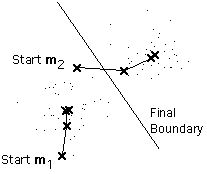# Study Apache Spark MLlib on IPython—Clustering—K-Means

HI Guys,

Today, let’s study the K-means, which is most commonly used clustering method.

### I. Clustering vs Classification

For those puzzling about difference between classification and clustering, (refer to)

Classification– The task of assigning instances to pre-defined classes. (Supervised)
–E.g. Deciding whether a particular patient record can be associated with a specific disease.

Clustering – The task of grouping related data points together without labeling them. (Unsupervised)
–E.g. Grouping patient records with similar symptoms without knowing what the symptoms indicate.II.

### II. Types of Clustering in MLlib

#### 1. Partitioning Approach

Clustering the dataset first and then use the same estimate standard

to converge

K-Means

#### 2. Model Based Approach

Estimate a distribution model and then find the best of it

GMM (Guassian Mixture Model)

LDA (Latent Dirichlet allocation—text processing)

#### 3. Dimensionality Reduction Approach

Reduce the dimensions the then clustering.

PIC (Power iteration clustering—–Graph processing)

#### 4. K-Means

Suppose that we have n example feature vectors x1, x2, …, xn all from the same class, and we know that they fall into k compact clusters, k < n. Let mi be the mean of the vectors in Cluster i. If the clusters are well separated, we can use a minimum-distance classifier to separate them. That is, we can say that x is in Cluster i if || xmi || is the minimum of all the k distances. This suggests the following procedure for finding the k means (reference):

• Make initial guesses for the means m1, m2, …, mk
• Until there are no changes in any mean
• Use the estimated means to classify the examples into clusters
• For i from 1 to k
• Replace mi with the mean of all of the examples for Cluster i
• end_for
• end_until1. Good and short tutorial about K-Means

2. scikit-learn K-means

3. Apache MLlib K-means

Let’s look at the IPython notebook. Github source codeWe can see these two models have a similar WSSSE(Within Set Sum of Squared Error) value. Scikit-learn gives 2470.602, whileas the MLlib result is 2450.403.

Reference,

1. https://spark.apache.org/docs/latest/mllib-clustering.html#k-means

3. http://www.cs.princeton.edu/courses/archive/fall08/cos436/Duda/C/k_means.htm